# Finding the Surface Area and Volume of Frustums of a Pyramid and Cone

• Author:
• Updated date:

Ray is a licensed engineer in the Philippines. He loves to write about mathematics and civil engineering.

## What Is a Frustum of a Right Circular Cone?

The frustum of a right circular cone is a portion of the cone enclosed by its base, a section that is parallel to the base, and the conical surface included between the base of the cone and the parallel section. A frustum of a circular cone has different parts. Listed below are the different elements of a frustum of a right circular cone.

1. Lower Base - a base of a frustum of a right circular cone with a larger radius

2. Upper Base - a base of a frustum of a right circular cone with a smaller radius

3. Altitude - the perpendicular distance between the bases of a frustum of a cone

4. Slant Height - the distance measured along the lateral face of the frustum

5. Radii -the distance from the center of a base to its edge

To determine the slant height (l) from the given radii of the bases and the altitude of the frustum, consider the projected trapezoid of the frustum to a plane. Using the Pythagorean Theorem, the equation will be:

l = √[h2 + (r2 - r1)2]

## Surface Area and Volume of a Frustum of a Right Circular Cone

The area of a conical surface of the frustum is the lateral surface area of the frustum. It is equal to one-half of the sum of the circumferences of the bases multiplied by the slant height. The variables C1 and C2 are the circumferences of the bases of the frustum, and l is the slant height. Since C1 = 2πr2 and C2 = 2πr2, replace C by 2πr. For the total surface area, of a frustum of a right circular cone is given by the sum of the lateral surface area and area of the two bases.

LSA = 1/2 (C1 + C2) (l)

LSA = π (r1 + r2) (l)

TSA = π (r1 + r2) (l) + πr12 + πr22

The volume of a frustum of a circular cone is equal to one-third of the sum of the two base areas and the square root of the two base areas, multiplied by the altitude. Since the two bases of a frustum of a cone are circles, you can substitute πr2 to the variable B resulting in a more specific equation of the volume.

Scroll to Continue

V = (1/3) (h) (B1 + B2 + √B1B2)

V = (1/3) (h) [πr12 + πr22 + √(πr12*πr22)]

V = (π/3) (h) (r12 + r22 + r1r2)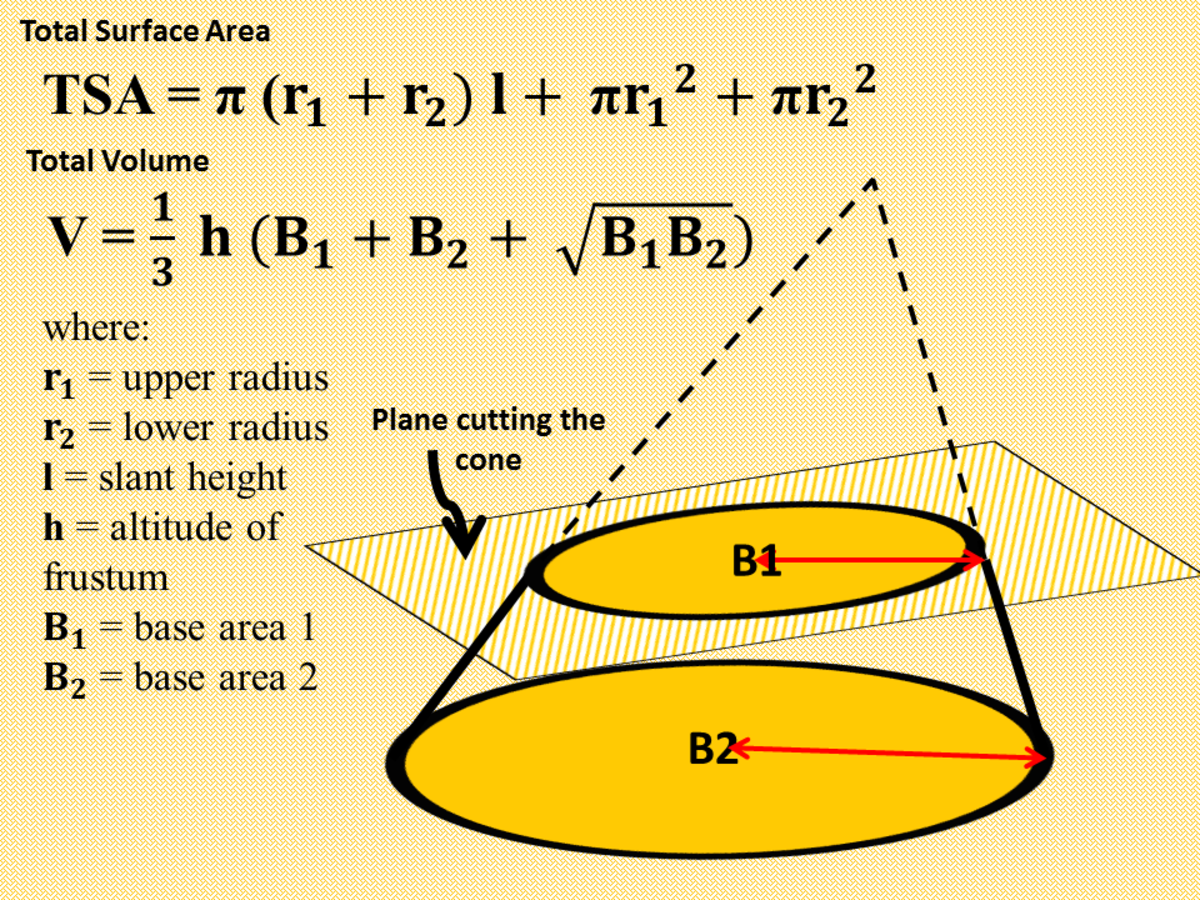Surface Area and Volume of Frustums of a Right Circular ConeJohn Ray Cuevas

## What Is a Frustum of a Regular Pyramid?

The frustum of a pyramid is a portion of a pyramid obtained by passing a plane parallel to the base, intersecting all the lateral edges. A frustum of a pyramid is a polyhedron enclosed by the lateral surface and the base of the pyramid. If the pyramid is a regular right pyramid, the frustum has congruent isosceles trapezoids as lateral faces. A frustum of a regular pyramid has different parts. Listed below are the different elements of a frustum of a regular pyramid.

1. Lower Base - a base of a frustum of a regular pyramid with a larger area

2. Upper Base - a base of a frustum of a regular pyramid with a smaller area

3. Altitude - the perpendicular distance between the bases of a frustum of a regular pyramid

4. Slant Height - the distance measured along the lateral face of the frustum

## Surface Area and Volume of a Frustum of a Regular Pyramid

The sum of the areas of the lateral faces of a frustum of a regular pyramid is the lateral surface area. Since each lateral face is an isosceles trapezoid, then the area of each lateral face is one-half of the sum of the two edge bases multiplied by the slant height. In the equation below, b1 and b2 are the edges of the upper base and lower base of a frustum of a regular pyramid. Since all the lateral faces are congruent, the lateral surface area of the frustum of a regular pyramid is equal to the number of sides of the base multiplied by the area of one trapezoid. In the equation below, P1 and P2 are the perimeters of the bases of the frustum. Lastly, the total surface area of the frustum is the sum of the lateral area and the areas of the two bases. B1 and B2 are the areas of the bases of the frustum.

A = 1/2 (b1 + b2) (l)

LSA = 1/2 (P1 + P2) (l)

TSA = LSA + B1 + B2

The volume of a frustum of a regular pyramid is equal to one-third of the altitude multiplied by the sum of its bases and the geometric mean between them.

V = 1/3 (h) (B1 + B2 + √(B1B2)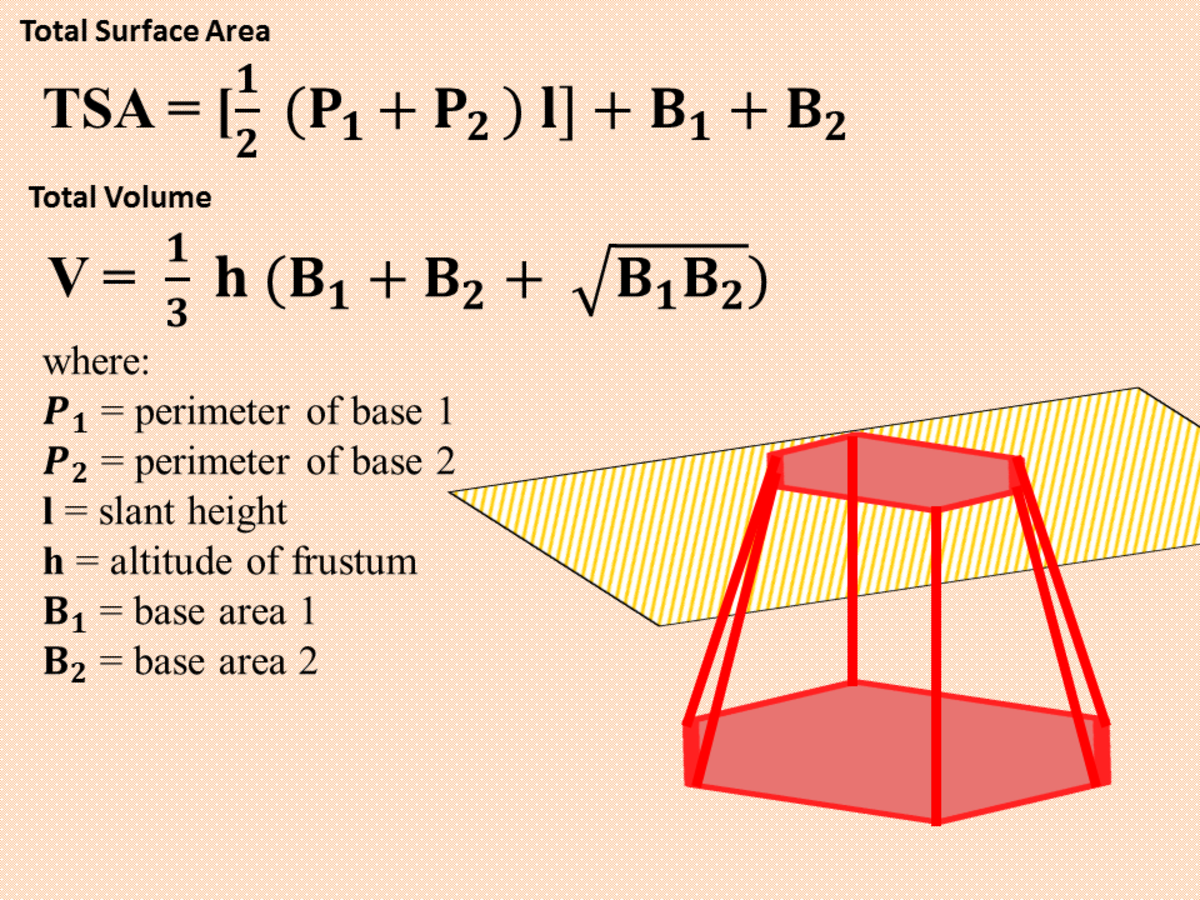Surface Area and Volume of Frustum of a Regular PyramidJohn Ray Cuevas

## Problem 1: Surface Area and Volume of Frustum of a Right Circular Cone

In a frustum of a right circular cone, the diameter of the lower base is 24 feet, while the diameter of the upper base is 14 feet. If the slant height of the frustum is 13 feet, find the total area and the volume of the frustum.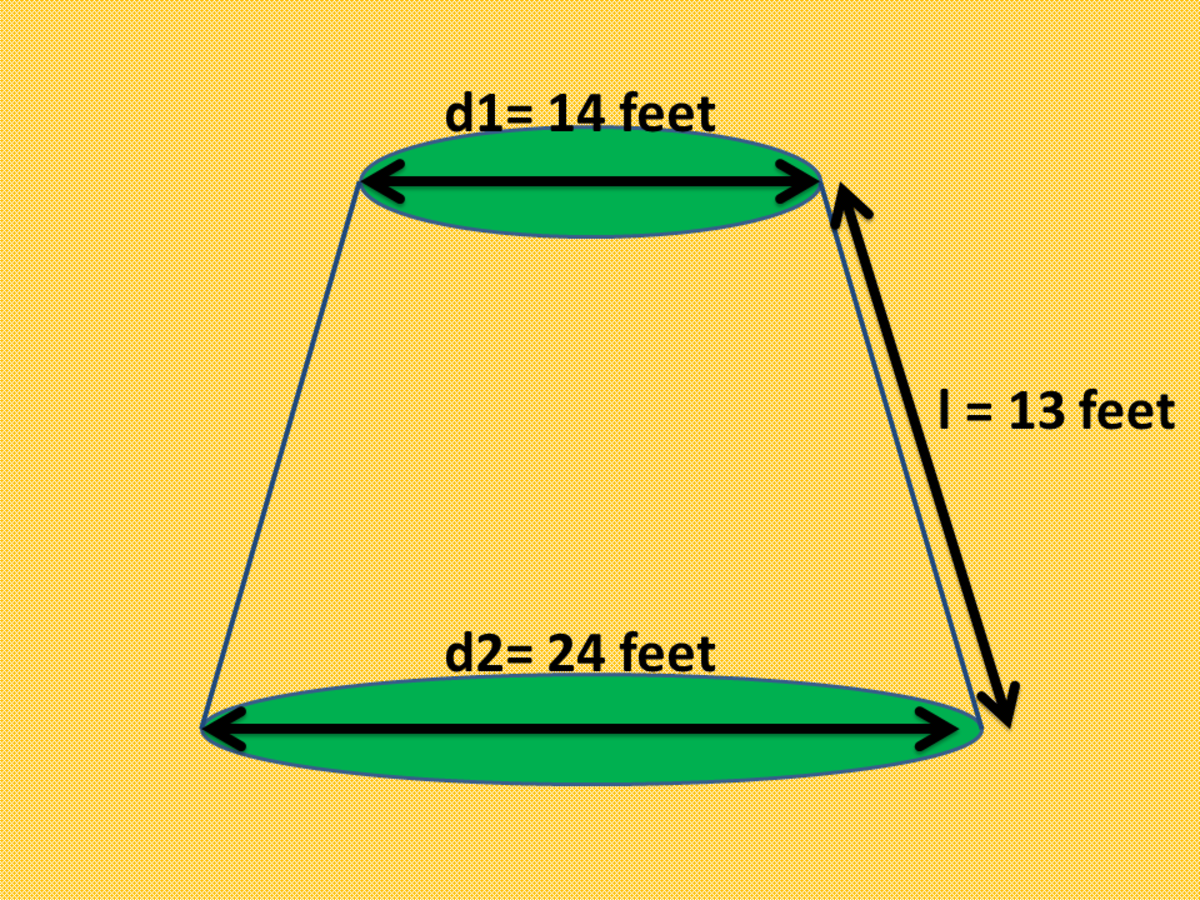How to Solve for the Area and Volume of Frustum of a Right Circular ConeJohn Ray Cuevas

### Solution

a. Given the diameter of the upper base d1 = 14 feet and diameter of the lower base d2 = 24 feet, compute for the two base areas using the formula for an area of a circle.

r1 = 14 / 2

r1 = 7 feet

r2 = 24 / 2

r2 = 12 feet

B1 = π (7)2

B1 = 49π ft2

B2 = π (12)2

B2 = 144π ft2

b. Solve for the height of the frustum of the right circular cone using the Pythagorean theorem.

h = √(c2 - a2)

h = √(132 - 52)

h = 12 feet

c. Compute the total surface area using the given equation.

TSA = B1 + B2 + π (r1 + r2) (l)

TSA = 49π + 144π + π (7 + 12) (13)

TSA = 1382 ft2

d. Compute the total volume of the frustum of the right circular cone given above.

V = (1/3) (h) (B1 + B2 + √B1B2)

V = (1/3) (12) (49π + 144π + √(49π)(144π)

V = 1108π ft3

V = 3481 ft3

Final Answer: The total surface area and volume of the frustum of the right circular cone given above are 1382 ft3 and 3481 ft3, respectively.

## Problem 2: Surface Area and Volume of Frustum of a Right Circular Cone

In a given frustum of a right circular cone, the radius of the lower base is 30 feet, while the radius of the upper base is 15 feet. If the altitude of the frustum is 20 feet, find the total surface area and volume of the frustum.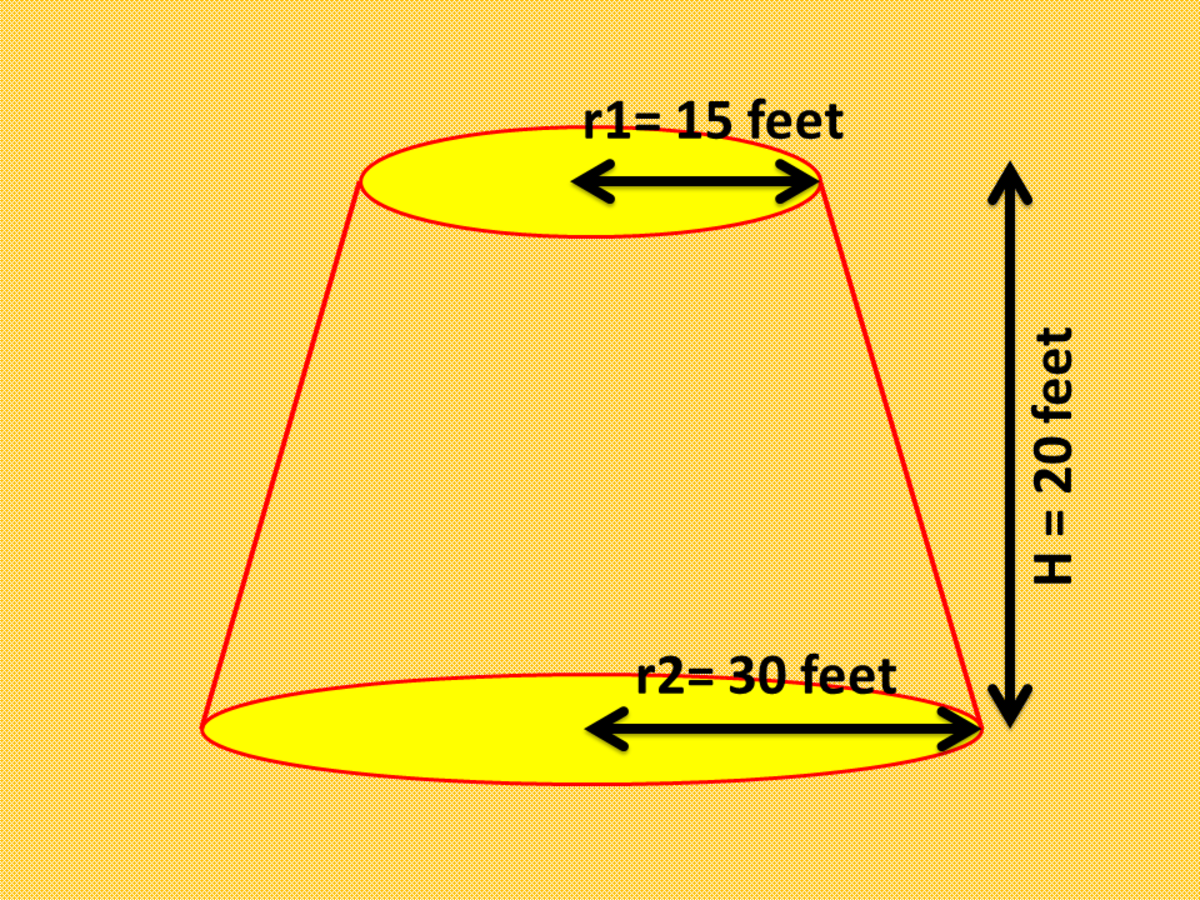How to Solve for the Area and Volume of Frustum of a Right Circular ConeJohn Ray Cuevas

### Solution

a. Given the radius of the upper base r1 = 15 feet and radius of the lower base r2 = 30 feet, compute for the two base areas using the formula for an area of a circle.

r1 = 15 feet

r2 = 30 feet

B1 = π (15)2

B1 = 225π ft2

B2 = π (30)2

B2 = 900π ft2

b. Solve for the slant height of the frustum of the right circular cone using the Pythagorean theorem.

l = √(b2 + a2)

l = √(152 + 202)

l = 25 feet

c. Compute the total surface area using the given equation.

TSA = B1 + B2 + π (r1 + r2) (l)

TSA = 225π + 900π + π (15 + 30) (25)

TSA = 7068.58 ft2

d. Compute the total volume of the frustum of the right circular cone given above.

V = (1/3) (h) (B1 + B2 + √B1B2)

V = (1/3) (20) (225π + 900π + √(225π)(900π)

V = 10500π ft3

V = 32986.72 ft3

Final Answer: The total surface area and volume of the frustum of the right circular cone given above are 7068.58 ft2 and 32986.72 ft3, respectively.

## Problem 3: Surface Area and Volume of Frustum of a Regular Pyramid

Find the volume and the total surface area of a frustum of a regular hexagonal pyramid with base edges of 8 centimeters and 10 centimeters, and an altitude of 10 centimeters.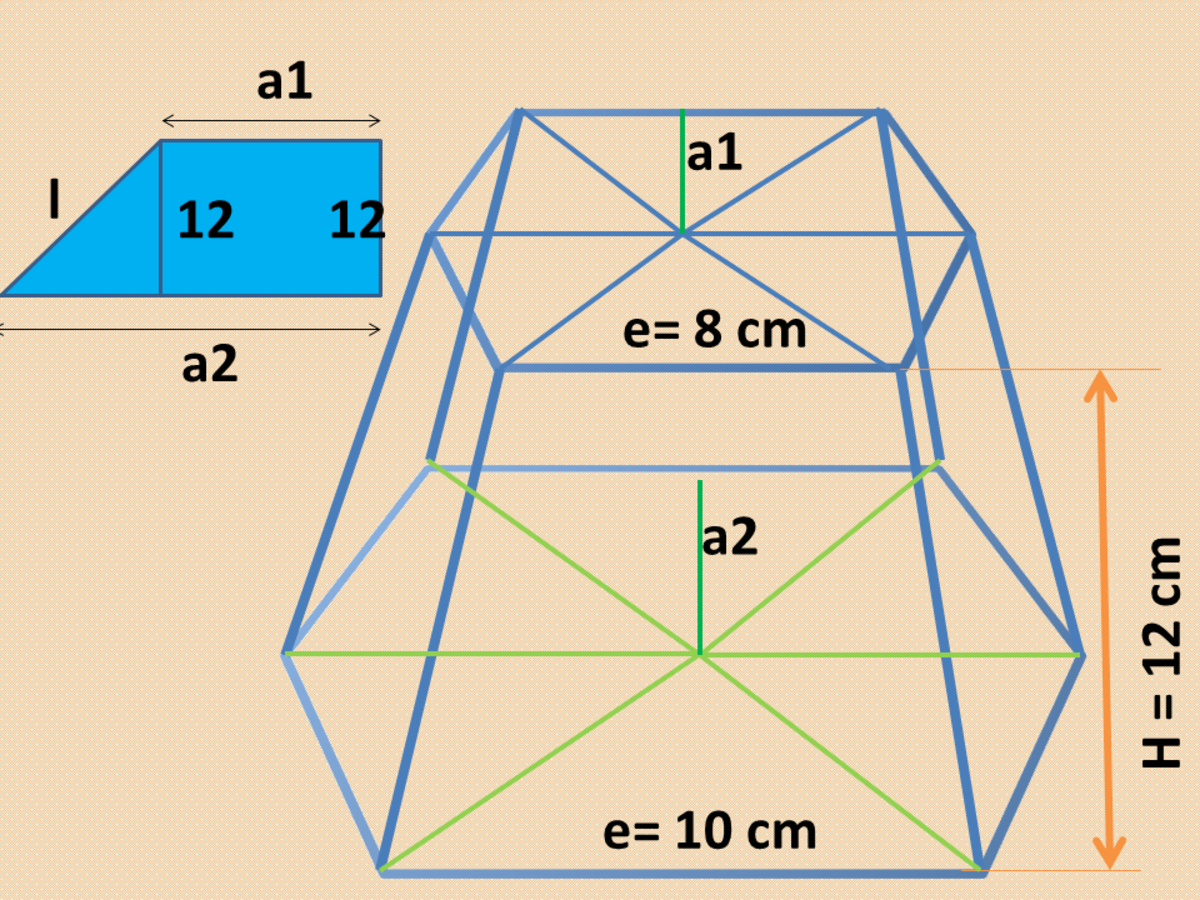How to Solve for the Area and Volume of Frustum of a Regular PyramidJohn Ray Cuevas

### Solution

a. Let l be the slant height of the given frustum, a1 the apothem of the upper base, and a2 the apothem of the lower base. Using the formula for finding the measure of the apothem given below, solve for a1 and a2.

a = (s/2) / (tan[180°/n])

a1 = (8/2) / (tan[180°/6])

a1 = 4√3 centimeters

a2 = (10/2) / (tan[180°/6])

a2 = 5√3 centimeters

b. In the trapezoidal figure shown above, solve for the value of the slant height.

l =√ (12)2 + (5√3 - 4√3)2

l = 7√3 centimeters

c. Compute for the total surface area by finding the lateral surface area first.

LSA = (1/2) (P1 + P2) (l)

LSA = (1/2) (6(8) + 6(10)) (7√3)

LSA = 654.72 cm2

d. Compute for the area of the two bases using the formula in finding the area of a regular polygon.

Barea = (ns2) / [4tan (180°/n)]

B1 = (6(8)2) / [4tan (180°/6)]

B1 = 96√3 cm2

B2 = (6(10)2) / [4tan (180°/6)

B2 = 259.81 cm2

e. Solve for the total surface area using the lateral surface area and area of the two bases obtained in previous steps.

TSA = B1 + B2 + LSA

TSA = 96√3 + 259.81 + 654.72

TSA = 1080.81 cm2

f. Compute for the volume of the frustum of the regular pyramid given above.

V = (1/3) (h) (B1 + B2 + √B1B2)

V = (1/3) (12) (96√3 + 259.81 + √(96√3)(259.81)

V = 2535.74 cm3

Final Answer: The total surface area and volume of the frustum of the regular pyramid given above are 1080.81 cm2 and 2535.74 cm3, respectively.

## Problem 4: Altitude of Frustum of a Regular Pyramid

Find the altitude of a frustum of a pyramid whose volume is 148 ft3.The base areas of the frustum are 9 ft3 and 36 ft3.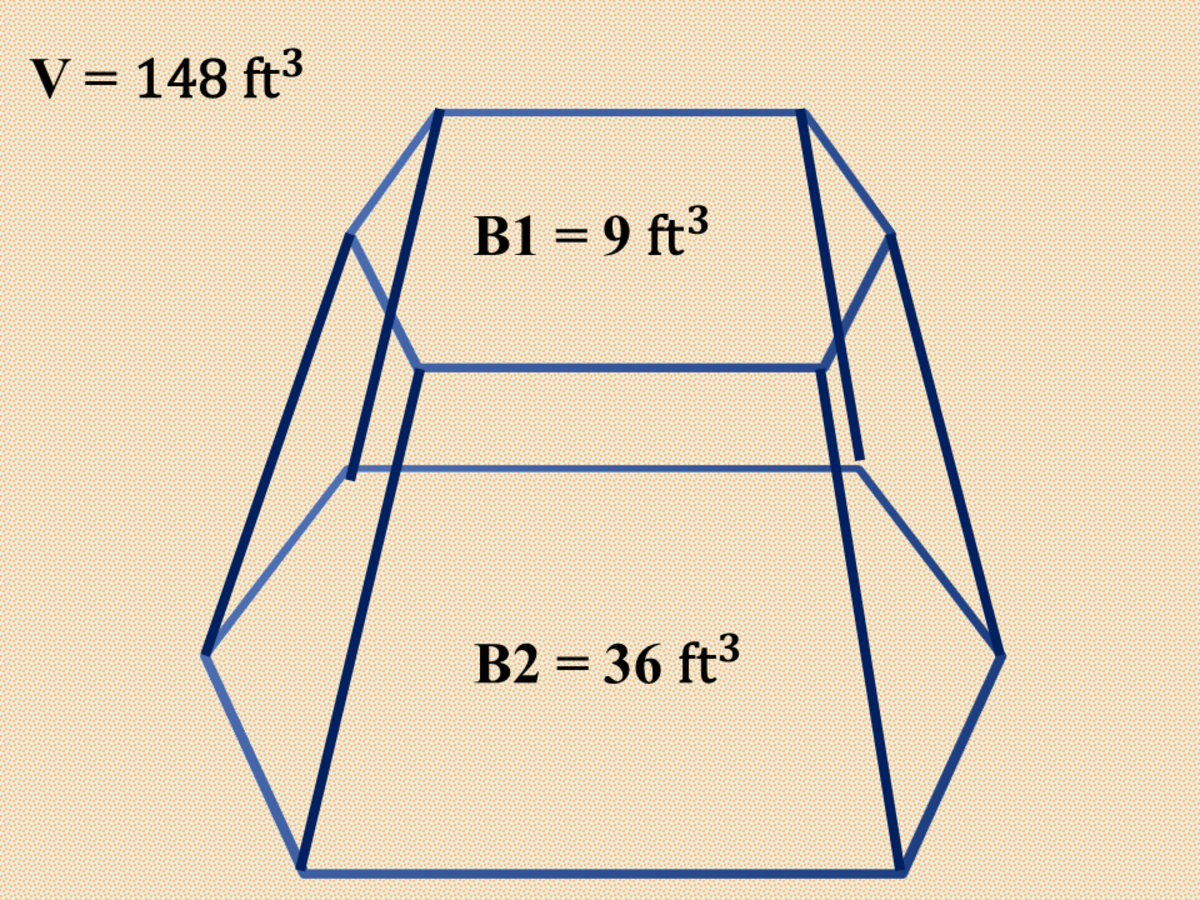How to Solve for the Area and Volume of Frustum of a Regular PyramidJohn Ray Cuevas

### Solution

a. In finding the altitude (h) of the given frustum of a pyramid, substitute the given values into the equation. Let V = 148 ft3, B1 = 9 ft2, and B2 = 36 ft2.

V = (1/3) (h) (B1 + B2 + √B1B2)

148 = (1/3) (h) (9 + 36 + √(9)(36)

h = 7.05 feet

Final Answer: The altitude of the given frustum of a regular pyramid is 7.05 feet.

## Problem 5: Lateral Area of Frustum of a Right Circular Cone

The volume of a right circular cone is 52π ft3. Its altitude is 3 feet and the measure of its lower radius is three times the measure of its upper radius. Find the lateral area of the frustum.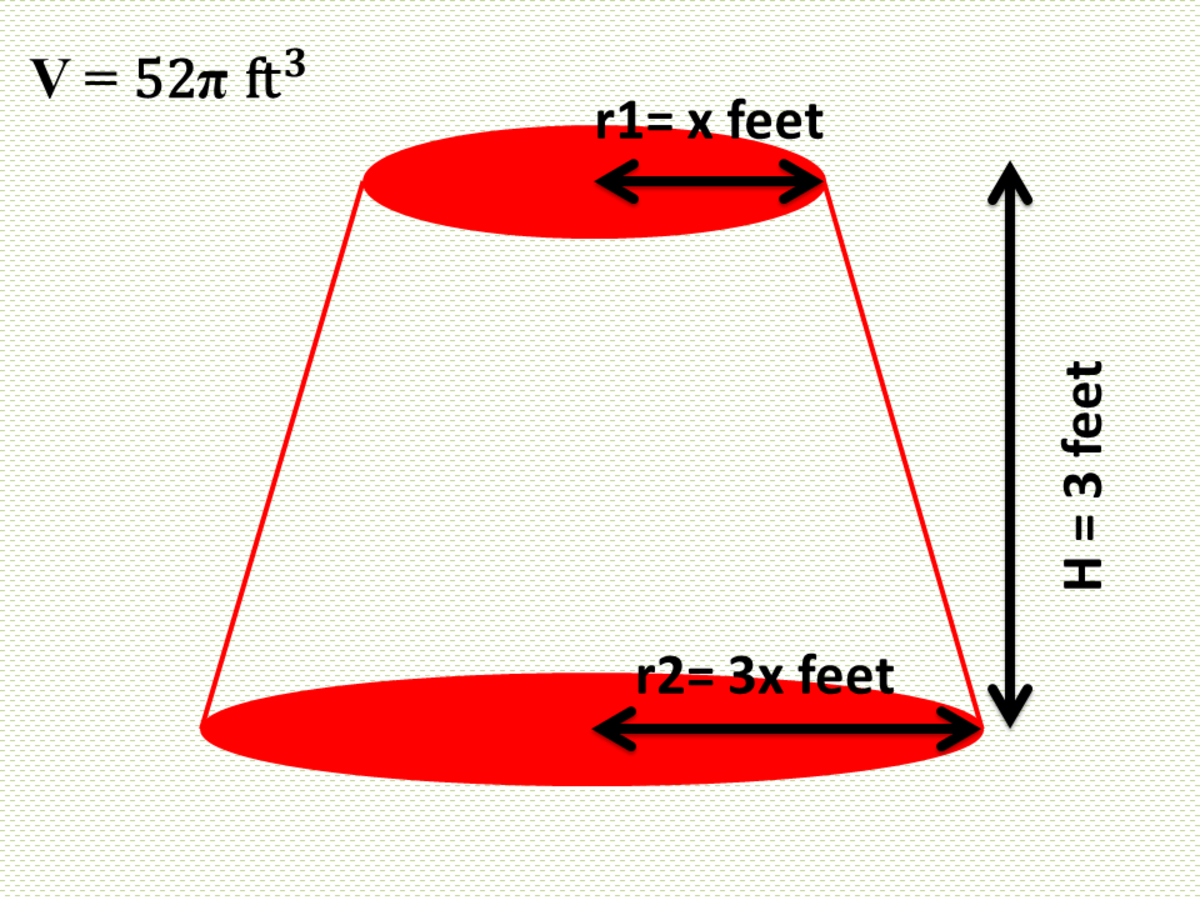Lateral Area of Frustum of a Right Circular ConeJohn Ray Cuevas

### Solution

a. Express the area of the two bases in terms of the variable x.
B1 = πr12
B1 = π(x)2
B2 = πr22

B2 = π(3x)2

B2 = π(9x2)

b. Given the volume of the right circular cone, compute for the value of x.

V = (1/3) (h) (B1 + B2 + √B1B2)

52π = (π/3) (3) (x2 + 9x2 + √(x2)(9x2)

x = 2 feet

r1 = 2 feet

r2 = 6 feet

c. Using Pythagorean theorem, compute for the slant height given the radius of the two bases and the altitude.

l = √[h2 + (r2 - r1)2]

l = √[32 + (6 - 2)2]

l = 5 feet

d. Solve for the lateral area using the given formula below.

LSA = π (r1 + r2) (l)

LSA = π (2 + 6) (5)

LSA = 40π ft2

Final Answer: The lateral surface area of the given frustum of a right circular cone is 40π ft2.